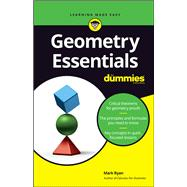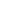# Geometry Essentials for Dummies

• ISBN13:

• ISBN10:

## 1119590442

• Format: Paperback
• Publisher: For Dummies

Note: Supplemental materials are not guaranteed with Rental or Used book purchases.

Purchase Benefits

•Free Shipping On Orders Over \$35!
Your order must be \$35 or more to qualify for free economy shipping. Bulk sales, PO's, Marketplace items, eBooks and apparel do not qualify for this offer.
•Get Rewarded for Ordering Your Textbooks! Enroll Now
List Price: \$9.99 Save up to \$1.60
• Rent Book \$8.99Free Shipping

TERM
PRICE
DUE
USUALLY SHIPS IN 3-4 BUSINESS DAYS
*This item is part of an exclusive publisher rental program and requires an additional convenience fee. This fee will be reflected in the shopping cart.

### Supplemental Materials

What is included with this book?

• The New copy of this book will include any supplemental materials advertised. Please check the title of the book to determine if it should include any access cards, study guides, lab manuals, CDs, etc.
• The Rental and eBook copies of this book are not guaranteed to include any supplemental materials. Typically, only the book itself is included. This is true even if the title states it includes any access cards, study guides, lab manuals, CDs, etc.

### Summary

Geometry Essentials For Dummies (9781119590446) was previously published as Geometry Essentials For Dummies (9781118068755). While this version features a new Dummies cover and design, the content is the same as the prior release and should not be considered a new or updated product.

Just the critical concepts you need to score high in geometry

This practical, friendly guide focuses on critical concepts taught in a typical geometry course, from the properties of triangles, parallelograms, circles, and cylinders, to the skills and strategies you need to write geometry proofs. Geometry Essentials For Dummies is perfect for cramming or doing homework, or as a reference for parents helping kids study for exams.

• Get down to the basics — get a handle on the basics of geometry, from lines, segments, and angles, to vertices, altitudes, and diagonals
• Conquer proofs with confidence — follow easy-to-grasp instructions for understanding the components of a formal geometry proof
• Take triangles in strides — learn how to take in a triangle's sides, analyze its angles, work through an SAS proof, and apply the Pythagorean Theorem
• Polish up on polygons — get the lowdown on quadrilaterals and other polygons: their angles, areas, properties, perimeters, and much more

### Author Biography

Mark Ryan is the owner of The Math Center in the Chicago area, where he teaches students in all levels of mathematics, from pre-algebra to calculus. He is the author of Calculus For Dummies and Geometry For Dummies.

Introduction 1

Conventions Used in This Book 2

Foolish Assumptions 2

Icons Used in This Book 3

Where to Go from Here 3

Chapter 1: An Overview of Geometry 5

The Geometry of Shapes 6

One-dimensional shapes 6

Two-dimensional shapes 6

Three-dimensional shapes 6

Geometry Proofs 6

Am I Ever Going to Use This? 7

When you’ll use your knowledge of shapes 7

When you’ll use your knowledge of proofs 8

Getting Down with Definitions 9

A Few Points on Points 11

Lines, Segments, and Rays 12

Horizontal and vertical lines 12

Doubling up with pairs of lines 13

Investigating the Plane Facts 14

Everybody’s Got an Angle 14

Five types of angles 15

Angle pairs 16

Bisection and Trisection 18

Segments 18

Angles 18

Chapter 2: Geometry Proof Starter Kit 21

The Lay of the (Proof) Land 21

Reasoning with If-Then Logic 23

If-then chains of logic 24

Definitions, theorems, and postulates 25

Bubble logic 26

Complementary and Supplementary Angles 27

Subtraction theorems 33

Like Multiples and Like Divisions 34

Congruent Vertical Angles 36

Transitivity and Substitution 37

Chapter 3: Tackling a Longer Proof 41

Making a Game Plan 42

Using All the Givens 42

Using If-Then Logic 43

Chipping Away at the Problem 45

Working Backward 47

Filling in the Gaps 49

Writing out the Finished Proof 49

Chapter 4: Triangle Fundamentals 51

Taking in a Triangle’s Sides 51

Scalene triangles 52

Isosceles triangles 52

Equilateral triangles 52

Triangle Classification by Angles 52

The Triangle Inequality Principle 53

Sizing up Triangle Area 54

A triangle’s altitude or height 54

Determining a triangle’s area 56

Regarding Right Triangles 57

The Pythagorean Theorem 58

Pythagorean Triple Triangles 60

The Fab Four triangles 61

Families of Pythagorean triple triangles 61

Two Special Right Triangles 64

The 45 - 45 - 90 triangle 64

The 30 - 60 - 90 triangle 66

Chapter 5: Congruent Triangle Proofs 69

Proving Triangles Congruent 69

SSS: The side-side-side method 70

SAS: Side-angle-side 72

ASA: The angle-side-angle tack 74

AAS: Angle-angle-side 74

Last but not least: HLR 75

Taking the Next Step with CPCTC 75

Defining CPCTC 76

Tackling a CPCTC proof 76

The Isosceles Triangle Theorems 79

The Two Equidistance Theorems 81

Determining a perpendicular bisector 81

Using a perpendicular bisector 83

Parallel Line Properties 85

Parallel lines with a transversal 85

The transversal theorems 87

Working with Auxiliary Lines 90

Properties of the parallelogram 93

Properties of the three special parallelograms 95

Properties of the kite 98

Properties of the trapezoid and the isosceles trapezoid 99

Proving That You’ve Got a Particular Quadrilateral 100

Proving you’ve got a parallelogram 100

Proving that you’ve got a rectangle, rhombus, or square 103

Proving that you’ve got a kite 104

Chapter 7: Polygon Formulas 107

Why the formulas work 108

Trying a few area problems 110

The Area of Regular Polygons 113

The polygon area formulas 114

Tackling an area problem 114

Angle and Diagonal Formulas 115

Interior and exterior angles 116

A polygon angle problem 117

Criss-crossing with diagonals 118

Chapter 8: Similarity 119

Similar Figures 119

Defining similar polygons 119

How similar figures line up 121

Solving a similarity problem 122

Proving Triangles Similar 124

Tackling an AA proof 125

Using SSS~ 126

An SAS~ proof 127

Splitting Right Triangles with the Altitude-on-Hypotenuse Theorem 128

More Proportionality Theorems 130

The Side-Splitter Theorem 130

The Angle-Bisector Theorem 132

Chapter 9: Circle Basics 135

Five circle theorems 136

Arcs and Central Angles 138

Tangents 138

The Pizza Slice Formulas 140

Determining arc length 140

Sector and segment area 141

The Angle-Arc Formulas 143

Angles on a circle 144

Angles inside a circle 144

Angles outside a circle 145

Keeping the formulas straight 146

The Power Theorems 147

The Chord-Chord Theorem 148

The Tangent-Secant Theorem 149

The Secant-Secant Theorem 149

Condensing the power theorems into a single idea 150

Chapter 10: 3-D Geometry 151

Flat-Top Figures 151

Pointy-Top Figures 154

Spheres 159

Chapter 11: Coordinate Geometry 161

The Coordinate Plane 161

Slope, Distance, and Midpoint 162

The slope dope 162

The distance formula 164

The midpoint formula 165

Trying out the formulas 166

Equations for Lines and Circles 167

Line equations 168

The circle equation 168

Chapter 12: Ten Big Reasons to Use in Proofs 171

The Reflexive Property 171

Vertical Angles are Congruent 171

The Parallel-Line Theorems 172

Two Points Determine a Line 172

If Sides, Then Angles 173

If Angles, Then Sides 173

Triangle Congruence 173

CPCTC 174

Triangle Similarity 174

Index 175